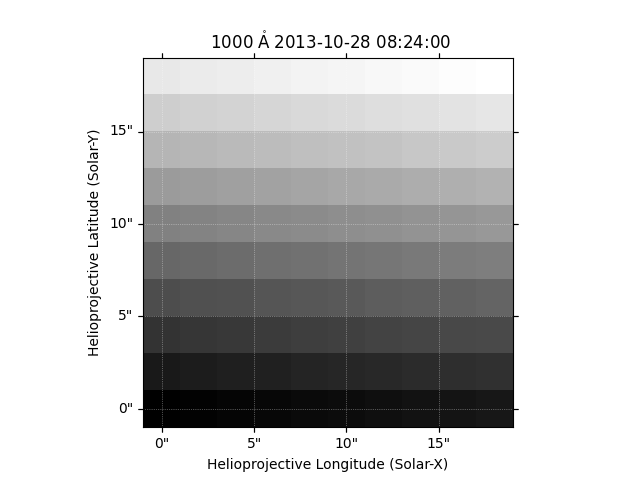# Generating a Map From Data¶

A simple demonstration of creating a map from a numpy array of data.

Start by importing the necessary modules.

from __future__ import print_function, division

import numpy as np
import matplotlib.pyplot as plt

import sunpy.map
import sunpy.data.sample


SunPy Maps store 2D data in a numpy array and additional data in a metadata dictionary giving information relating to the data and instrument.

data = np.random.rand(20,15)
header = {}
manual_map = sunpy.map.Map((data, header))


In general the attributes are populated using details in the metadata and in this case there is no centre pixel or pixel size information given so SunPy is defaulting to assuming each pixel is 1 arcsec.

print(manual_map.xrange)
print(manual_map.yrange)
# This is in Helioprojective tangent projection in both longitude and latitude:
print(manual_map.coordinate_system)


Out:

[-7.  8.] arcsec
[-9.5 10.5] arcsec
SpatialPair(axis1='HPLN-   ', axis2='HPLT-   ')


You can quickly plot a map using the peek method:

manual_map.peek()
plt.show()Total running time of the script: ( 0 minutes 0.473 seconds)

Gallery generated by Sphinx-Gallery